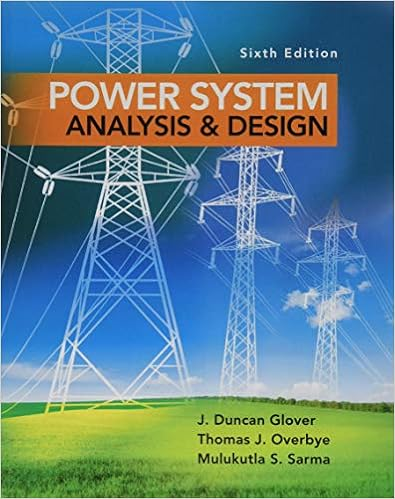# A bfo is used in demodulation of which types of s

• Test Prep
• 123
• 100% (5) 5 out of 5 people found this document helpful

This preview shows page 37 - 40 out of 123 pages.

##### We have textbook solutions for you!
The document you are viewing contains questions related to this textbook.The document you are viewing contains questions related to this textbook.
Chapter 5 / Exercise 5.33
Power System Analysis and Design
Glover/OverbyeExpert Verified
37. A BFO is used in demodulation of which types of signals? a) AMb) FMa) 1.6 MHz b) 19 MHzc) 19.6 MHzd) 21.2 MHz42. The key conceptual circuit in a superhet receiver is thea) mixerb) RF Amplifier c) IF Amplifier d) AF Amplifier
##### We have textbook solutions for you!
The document you are viewing contains questions related to this textbook.The document you are viewing contains questions related to this textbook.
Chapter 5 / Exercise 5.33
Power System Analysis and Design
Glover/OverbyeExpert Verified
43. A tuned circuit is resonant at 4 MHz, it’sQ is 100. The bandwidth isa) 100 kHz b) 4 kHzc) 40 kHzd) 400 kHza) Balancedb) Asymmetric c) Symmetric d) Both a and c 48. The current ratio of an attenuator is 5. Therefore its power ratio is .44. A crystal filter has a 6 dB bandwidth ofa)5 2.6 kHz and a 60 dB bandwidth of 14 kHz.b)10 The shape factor isc) 20 d)25a) 0.186 b) 5.38 c) 8.3 d) 36.4 45. Which noise figure represents the lowest noise? a) 1.6dBb) 2.1dB49. At resonance, the circuit impedance isa) inductive b) capacitive c) complex d) Resistive50. A band reject filter is composed of ac) 2.7dBd) 3.4dB46. At resonance, the power factor is a) 0 b) 1 c) <1 d) >147. A pi pad is a network. LPF connected inHPF. a) seriesb) shuntc) either a or b d) neither a or bwith a
COMMUNICATIONS IFinal Exam (4TH Quarter 2007 2008)1. The overall power gain of three amplifiers 8. The equivalent noise temperature of an in cascade is 46 dB. Their individual gains amplifier is 25 ºK. What is the noise factor? are: 100, 80 and . a. 0.913 a. 4.98 b. 0.086 b. 0.0249 c. 1.086 c. 5.65 d. 10.086 d. 60 9. This is also called modulation noise. 2. The tuned circuit has a Bandwidth equal to a. shot noise 400 KHz, if Q is 16, what is the resonant b. solar noise frequency? c. flicker noise a. 416 KHz b. 6.4 MHz c. none of the choices d. 25 MHz 3. A passive low pass filter having a cut-off frequency of 15 kHz will need what capacitance value if the resistance is equal to 1 KΩ?a. 106.1 nF b. 10.61 nF c. 1.061 nF d. 0.161 nF 4. This is a type of filter that passes all frequencies within a band between a lower and an upper critical frequency and rejects all others outside the band. a. band pass filter b. band reject filter c. high pass filter d. low pass filter 5. The most efficient RF power amplifier is the a. Class A b. Class B c. Class AB d. Class C 6. A parallel LC tuned circuit has a coil of 6 μH and 55pF capacitor. The coil resistance is 17 Ω,find Q a. 20.3 b. 19.4 c. 8.7 d. none of the choices 7. Which oscillators are preferred for carrier generators because of their good frequency stability? a. LC b. RC c. LR d. Crystal d. thermal noise 10. Most internal noise comes from a. shot noise b. transit time noise c. skin effect d. thermal agitation
•••